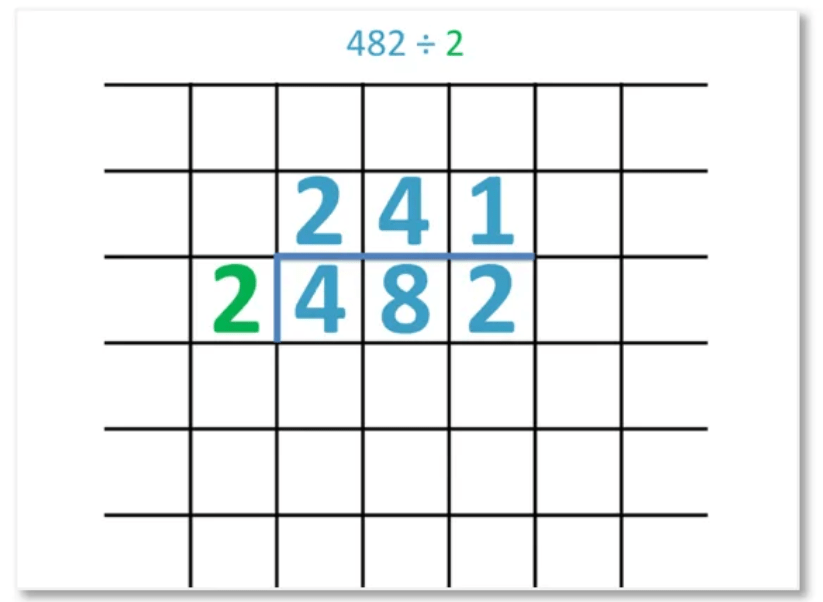# How to Find the Lowest Common Multiple

How to Find the Lowest Common Multiple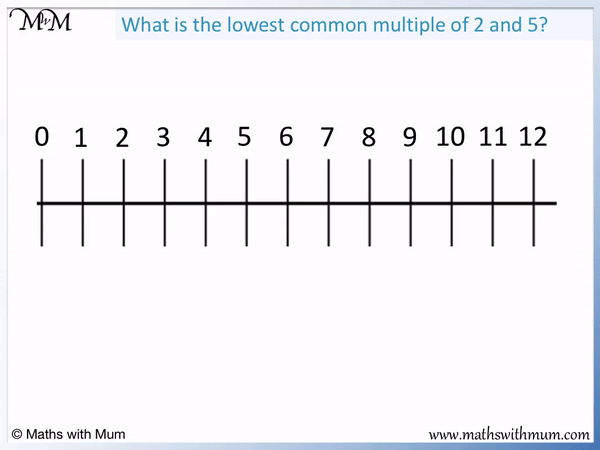• The lowest common multiple (LCM) is the first number that is a multiple of all of the numbers given. It is also known as the least common multiple.
• List the times tables of each number.
• Stop the list when you get to the value of the two numbers multiplied together. 2 × 5 = 10, so we stop at 10.
• The 2 times table is 2, 4, 6, 8, 10.
• The 5 times table is 5 and 10.
• The lowest common multiple is the first number to appear in both lists.
• The lowest common multiple of 2 and 5 is 10.
The lowest common multiple is the first number to appear in all times tables of the numbers given.• To find the least common multiple, list the multiples of each number and write down the number that appears in both lists.
• We only need to list up to the product of the two numbers. 4 × 6 = 24, so we can stop at 24.
• The 4 times table is 4, 8, 12, 16, 20, 24.
• The 6 times table is 6, 12, 18, 24.
• 12 is the first number to appear in both lists and so the least common multiple of 4 and 6 is 12.
• Even though 24 also appears in both lists, it is not the least common multiple because it is not the first number to do so.Supporting Lessons# Finding the Lowest Common Multiple

## What is the Least Common Multiple?

The least common multiple, or lowest common multiple, is the first number to appear in the list of multiples of all of the given numbers. For example, the least common multiple of 2 and 5 is 10 because it is the first number to appear in both the 2 and 5 times table.

The least common multiple (lowest common multiple) is commonly referred to as the LCM for short.

A multiple is just a number in the times tables of another number.

The multiples of 2 are 2, 4, 6, 8, 10 and so on.

The multiples of 5 are 5, 10, 15, 20 and so on.

The lowest common multiple is the lowest multiple that is in common to both numbers.

The multiples of 2 and 5 are shown below.The first number in both lists of multiples is 10. We say that 10 is the lowest common multiple of 2 and 5.The least common multiple is used in lots of other branches of mathematics. For example, the lowest common multiple is used when adding and subtracting fractions. The denominator of two fractions is changed to the least common multiple of both denominators before adding them. This is called finding the least common denominator.

Here is an example of using the least common multiple in a real life problem. Burgers are sold in packets of 5 and buns are sold in packets of 6. A burger is needed for each bun. What is the smallest number of burgers and buns that should be bought so that there are no left over burgers or buns?

To solve this real life problem, we need to find the least common multiple. We want the first number in both the 5 and 6 times table. The multiples of 5 are: 5, 10, 15, 20, 25, 30. The multiples of 6 are: 6, 12, 18, 24, 30. The first number to appear in both times tables is 30. We need 30 burgers and 30 buns.

In simple terms, the least common multiple means the first number to appear in all of the times tables of the numbers given.

## How to Calculate the Least Common Multiple

To calculate the least common multiple, follow these steps:

1. Multiply the numbers together.
2. List the multiples of each number up to this value.
3. The least common multiple is the smallest number that appears in all of the lists.

For example, find the lowest common multiple of 4 and 6.

The first step is to multiply the numbers together.

4 × 6 = 24. We already know that 24 is in both of the 4 and 6 times tables and so, we don’t need to look at numbers that are larger than this.

The second step is to list the multiples of each number.

We only need to list the multiples up to 24.

The multiples of 4 are 4, 8, 12, 16, 20 and 24.

The multiples of 6 are 6, 12, 18 and 24.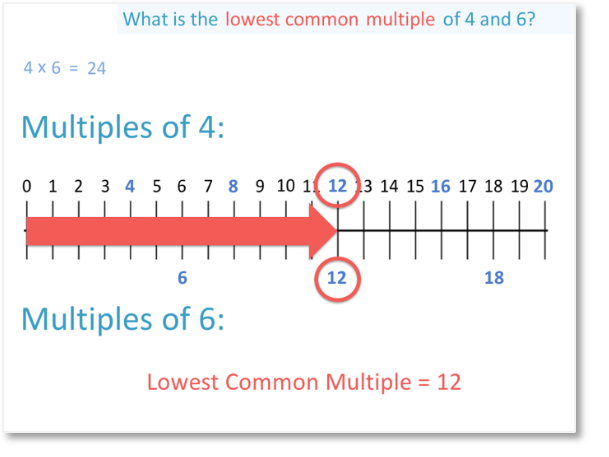The final step is to find the smallest number that appears in both lists.

The first number to appear in both lists is 12.12 is the least common multiple of 4 and 6. This means that 12 is the first number that is in both the 4 and the 6 times table.

Here is another example of finding the lowest common multiple of 3 and 5.

The first step is to multiply the numbers. 3 × 5 = 15 and so, we only need to list multiples up to 15.

The multiples of 3 are 3, 6, 9, 12 and 15.

The multiples of 5 are 5, 10 and 15.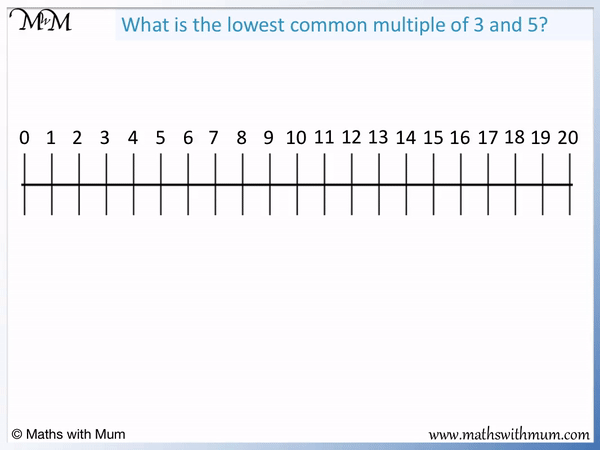The next step is to find the first number that is in both lists.

15 is the only number in both lists of multiples and so, 15 is the lowest common multiple of 3 and 5.

In this example, we can see that the least common multiples is simply 3 × 5.

If all of the numbers are prime, then their least common multiple is found by multiplying the numbers together.

Here is another example of finding the lowest common multiple of 2 and 10.

The multiples of 2 are 2, 4, 6, 8, 10, 12, 14, 16, 18 and 20.

The multiples of 10 are 10 and 20.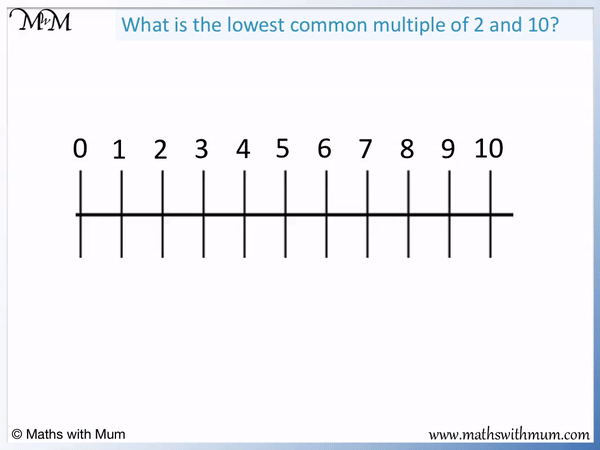The lowest common multiple of 2 and 10 is 10.

If the other numbers can divide exactly into the larger number, the larger number is the least common multiple.

10 is a multiple of 2 and so, 10 is the LCM of 2 and 10.

## How to Find the Least Common Multiple of 3 Numbers

To find the least common multiple of 3 numbers, follow these steps:

List the multiples of each number.
1. The least common multiple is the smallest number that appears in all 3 lists.

For example, find the LCM of the 3 numbers of 3, 5 and 6.

The first step is to list the multiples of each number.

The first few multiples of 3 are 3, 6, 9, 18, 21, 24, 27, 30, 33 and 36.

The first few multiples of 5 are 5, 10, 15, 20, 25, 30, 35, 40.

The first few multiples of 6 are 6, 12, 18, 24, 30, 36, 42.The next step is to find the smallest number that is in all of the lists.

30 is the first number to appear in the multiples of 3, 5 and 6.

The lowest common multiple of 3, 5 and 6 is 30.

Finding the lowest common multiple of more numbers is done in the same way as it is for 2 or 3 numbers. To find the lowest common multiple, simply list the multiples of all of the numbers and then find the first number that is in all of the lists.Now try our lesson on Short Division without Remainders where we introduce the short division method.